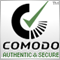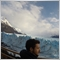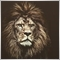# safeguarding scripts: exporting functions via TCP/web request at runtime251

I'm developing some proprietary strategies & i don't want to keep everything in the mql/ex4 file. I fear it can get decompiled sooner or later.

From what i've heard, function headers/details can be read from memory. Dll-only solutions are not safe either, as dll can be decoded.

SO i'm thinking about a system that will export some codes/functions from remote server. (before init)

I'm aware that incoming/outgoing get/post stuff can be caught via network sniffers. But i'll encode-decode all incoming & outgoing packages. So it's not a concern.

A sketch of the concept: (maybe some of you can give a better idea)

```#import TCP.dll//or Inet
string importedFunction(string result)
#

int init()
{
importedFunction("test message");

}

////////////////////////////////////////
//importedFunction() resides in a remote server
//////////////////////////////////////////

string importedFunction(string result){return result;}```5587

Tusher Ahmed:

I'm developing some proprietary strategies & i don't want to keep everything in the mql/ex4 file. I fear it can get decompiled sooner or later.

From what i've heard, function headers/details can be read from memory. Dll-only solutions are not safe either, as dll can be decoded.

SO i'm thinking about a system that will export some codes/functions from remote server. (before init)

I'm aware that incoming/outgoing get/post stuff can be caught via network sniffers. But i'll encode-decode all incoming & outgoing packages. So it's not a concern.

A sketch of the concept: (maybe some of you can give a better idea)

Your remote server could be hacked too...

I think keeping it simple is better, so you can focus only on your strategies improvements. If someone really wants your code anyway and has skills or money to pay some skilled guys, prob you are doomed, even if you are a pro in security servers... MetaQuotes has a team concerned in security measures and they have a huge business model that depends on that and in MQL5 editor now you can compile your code with extra security.

This is just my opinion.

Best regards,

Romeu Bertho.251

Romeu Bertho:

Your remote server could be hacked too...

I think keeping it simple is better, so you can focus only on your strategies improvements. If someone really wants your code anyway and has skills or money to pay some skilled guys, prob you are doomed, even if you are a pro in security servers... MetaQuotes has a team concerned in security measures and they have a huge business model that depends on that and in MQL5 editor now you can compile your code with extra security.

This is just my opinion.

Best regards,

Romeu Bertho.

yes you are right MetaEditor already provides some great level of security. However from my experience, old version of mql codes get hacked 1/2years later .

See all scripts from 3-4 years ago are now hacked. Some groups are providing decompilers for that aswell/20713

Tusher Ahmed: See all scripts from 3-4 years ago are now hacked. Some groups are providing decompilers for that aswell/

There has be zero proof that any ex4/5 can be decompiled since February 3, 2014 (Build 600)
Upcoming MetaTrader 4 and MQL4 Upgrades - Big Changes Are Underway (MetaQuotes Software Corp.) - MQL4 and MetaTrader 4 - MQL4 programming forum
Code Protection: New MQL4 language(Build 600+) decompilation protection and other crack techniques. (Macos Silva) - MQL4 and MetaTrader 4 - MQL4 programming forum - Page 2251

Here's a recent example from a client asking me to work on this hacked script : (look at the identifiers & signatures--it has got to be hacked by the decompiler). On average i complete 5/7 projects per week &   I'm seeing such decompiled scripts (both new & old) every now & then.

```/*
Generated by EX4-TO-MQ4 decompiler V4.0.277.4 []
Website: http://purebeam.biz
E-mail : purebeam@gmail.com
*/

#property indicator_chart_window
#property indicator_buffers 2
#property indicator_color1 Red
#property indicator_color2 Olive

int gi_76 = 6;
int gi_80 = 500;
extern bool SoundAlarm = True;
extern bool EmailAlarm = True;
double g_ibuf_92[];//for bull
double g_ibuf_96[];//for bear
int gi_100 = 0;
int gi_104 = 0;

int init() {
IndicatorBuffers(2);
SetIndexStyle(0, DRAW_ARROW);
SetIndexArrow(0, 234);
SetIndexStyle(1, DRAW_ARROW);
SetIndexArrow(1, 233);
SetIndexBuffer(0, g_ibuf_92);
SetIndexBuffer(1, g_ibuf_96);
GlobalVariableSet("AlertTime" + Symbol() + Period(), TimeCurrent());
GlobalVariableSet("SignalType" + Symbol() + Period(), 5);
return (0);
}

int deinit() {
GlobalVariableDel("SignalType" + Symbol() + Period());
return (0);
}

int start() {
int li_12;
double ld_52;
double ld_60;
double ld_68;
double ld_76;
double ld_84;
double ld_92;
double ld_100;
double lda_108;
double ld_120;
if (gi_80 >= 1000) gi_80 = 950;
SetIndexDrawBegin(0, Bars - gi_80 + 11 + 1);
SetIndexDrawBegin(1, Bars - gi_80 + 11 + 1);
int l_ind_counted_8 = IndicatorCounted();
double ld_112 = 0;
int li_20 = gi_76 * 2 + 3;
double ld_36 = gi_76 + 67;
double ld_44 = 33 - gi_76;
int l_period_24 = li_20;
if (Bars <= 12) return (0);
if (l_ind_counted_8 < 12) {
for (int li_0 = 1; li_0 <= 0; li_0++) g_ibuf_92[gi_80 - li_0] = 0.0;
for (li_0 = 1; li_0 <= 0; li_0++) g_ibuf_96[gi_80 - li_0] = 0.0;
}
for (int li_4 = gi_80 - 11 - 1; li_4 >= 0; li_4--) {
li_12 = li_4;
ld_76 = 0.0;
ld_84 = 0.0;
for (li_12 = li_4; li_12 <= li_4 + 9; li_12++) ld_84 += MathAbs(High[li_12] - Low[li_12]);
ld_76 = ld_84 / 10.0;
li_12 = li_4;
ld_68 = 0;
while (li_12 < li_4 + 9 && ld_68 < 1.0) {
if (MathAbs(Open[li_12] - (Close[li_12 + 1])) >= 2.0 * ld_76) ld_68 += 1.0;
li_12++;
}
if (ld_68 >= 1.0) ld_92 = li_12;
else ld_92 = -1;
li_12 = li_4;
ld_68 = 0;
while (li_12 < li_4 + 6 && ld_68 < 1.0) {
if (MathAbs(Close[li_12 + 3] - Close[li_12]) >= 4.6 * ld_76) ld_68 += 1.0;
li_12++;
}
if (ld_68 >= 1.0) ld_100 = li_12;
else ld_100 = -1;
if (ld_92 > -1.0) l_period_24 = 3;
else l_period_24 = li_20;
if (ld_100 > -1.0) l_period_24 = 4;
else l_period_24 = li_20;
ld_52 = 100 - MathAbs(iWPR(NULL, 0, l_period_24, li_4));
lda_108[li_4] = ld_52;
g_ibuf_92[li_4] = 0;
g_ibuf_96[li_4] = 0;
ld_60 = 0;
if (ld_52 < ld_44) {
for (int li_16 = 1; lda_108[li_4 + li_16] >= ld_44 && lda_108[li_4 + li_16] <= ld_36; li_16++) {
}
if (lda_108[li_4 + li_16] > ld_36) {
ld_60 = High[li_4] + ld_76 / 2.0;
if (li_4 == 1 && gi_100 == FALSE) {
gi_100 = TRUE;
gi_104 = FALSE;
}
g_ibuf_92[li_4] = ld_60;
}
}
if (ld_52 > ld_36) {
for (li_16 = 1; lda_108[li_4 + li_16] >= ld_44 && lda_108[li_4 + li_16] <= ld_36; li_16++) {
}
if (lda_108[li_4 + li_16] < ld_44) {
ld_60 = Low[li_4] - ld_76 / 2.0;
if (li_4 == 1 && gi_104 == FALSE) {
gi_104 = TRUE;
gi_100 = FALSE;
}
g_ibuf_96[li_4] = ld_60;
}
}
}
if (gi_100 == TRUE && TimeCurrent() > GlobalVariableGet("AlertTime" + Symbol() + Period()) && GlobalVariableGet("SignalType" + Symbol() + Period()) != 0.0) {
ld_120 = High[iHighest(Symbol(), 0, MODE_HIGH, 3, 0)] + 5.0 * Point;
if (SoundAlarm) Alert("Sell signal @ ", Symbol(), " Period ", Period(), " set-Stop Loss @", ld_120);

if (EmailAlarm) SendMail("Sell Signal FX Marksman", "Sell signal @ " + Symbol() + " Period " + Period() + " Stop Loss @ " + ld_120);
if(Send_Push_Notification)SendNotification("Sell Signal FX Marksman"+ "Sell signal @ " + Symbol() + " Period " + Period() + " Stop Loss @ " + ld_120);
ld_112 = TimeCurrent() + 60.0 * (Period() - MathMod(Minute(), Period()));
GlobalVariableSet("AlertTime" + Symbol() + Period(), ld_112);
GlobalVariableSet("SignalType" + Symbol() + Period(), 0);
}
if (gi_104 == TRUE && TimeCurrent() > GlobalVariableGet("AlertTime" + Symbol() + Period()) && GlobalVariableGet("SignalType" + Symbol() + Period()) != 1.0) {
ld_120 = Low[iLowest(Symbol(), 0, MODE_LOW, 3, 0)] - 5.0 * Point;
if (SoundAlarm) Alert("Buy signal @ ", Symbol(), " Period ", Period(), " set-Stop Loss @", ld_120);
if (EmailAlarm) SendMail("BUY Signal FX Marksman", "Buy signal @ " + Symbol() + " Period " + Period() + " Stop Loss @ " + ld_120);
if(Send_Push_Notification)SendNotification("BUY Signal FX Marksman"+ "Buy signal @ " + Symbol() + " Period " + Period() + " Stop Loss @ " + ld_120);
ld_112 = TimeCurrent() + 60.0 * (Period() - MathMod(Minute(), Period()));
GlobalVariableSet("AlertTime" + Symbol() + Period(), ld_112);
GlobalVariableSet("SignalType" + Symbol() + Period(), 1);
}
return (0);
}
```9911

Tusher Ahmed:

Here's a recent example from a client asking me to work on this hacked script : (look at the identifiers & signatures--it has got to be hacked by the decompiler). On average i complete 5/7 projects per week &   I'm seeing such decompiled scripts (both new & old) every now & then.

This is an old one